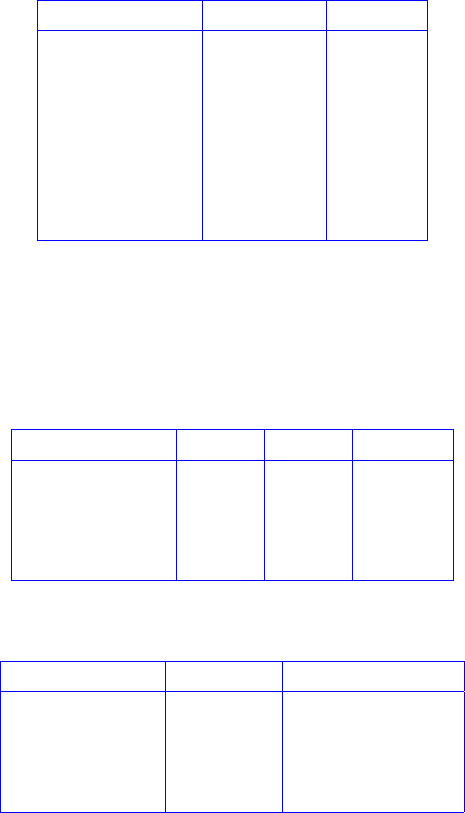Get 2 days of premium access
Study Guides (380,000)
US (220,000)
Berkeley (5,000)
COMPSCI (500)
Quiz

# COMPSCI 70 Study Guide - Quiz Guide: Alistair Sinclair, Prime Number, Prime FactorExam

Department
Computer Science
Course Code
COMPSCI 70
Professor
Rao Satish
Study Guide
Quiz

This preview shows pages 1-2. to view the full 7 pages of the document.CS 70 Discrete Mathematics and Probability Theory
Fall 2018 Alistair Sinclair and Yun Song HW 4
1 Modular Arithmetic Solutions
Find all solutions (modulo the corresponding modulus) to the following equations. Prove that there
are no other solutions (in a modular setting) to each equation.
(a) 2x5(mod 15)
(b) 2x5(mod 16)
(c) 5x10 (mod 25)
Solution:
(a) Since 2 has an inverse modulo 15, this equation will have exactly one solution. We can get this
solution by multiplying both sides by 21(mod 15), which is 8. This gives us that x40 10
(mod 15).
(b) Our trick from the last part doesn’t work any more, since 2 is not relatively prime to 16, and
thus doesn’t have a multiplicative inverse mod 16. Indeed, this equation has no solutions at all,
since the left side will always be even and the right side will always be odd. More formally,
we know that 2x5(mod 16)is equivalent to 2x+16k=5 for some kZ. We can factor
out a two from the left hand side, showing that it is divisible by 2, so there is no choice of x
and kthat will make the equality hold.
(c) Again, we have that the coefﬁcient on xdoes not have a multiplicative inverse in our chosen
modulus. However, unlike the previous part, we can still ﬁnd solutions to this equation. We
can see by inspection that x=2 will be one possible solution. Furthermore, if xis a solution
to this equation, x+5 will be as well, since 5(x+5) = 5x+25 5x(mod 25). This tells us
that x=7, x=12, x=17, and x=22 are also solutions to this equation.
We now just have to show that these are the only ﬁve solutions to this equation modulo 25.
Suppose we have some solution xto the equation. This means that 5x10 (mod 25), or
equivalently, that 5x+25k=10 for some kZ. This is now an equation over the real num-
bers, so we can divide the whole thing by 5, giving us that x+5k=2. But now that’s just
deﬁnitionally saying that x2(mod 5). Thus, we have that all solutions to this equation
must be equivalent to 2 modulo 5–and the only numbers modulo 25 that satisfy this condition
are 2, 7, 12, 17, and 22. This tells us that indeed, these ﬁve numbers are the only possible
values for x.
CS 70, Fall 2018, HW 4 1

Only pages 1-2 are available for preview. Some parts have been intentionally blurred.2 Euclid’s Algorithm
(a) Use Euclid’s algorithm from lecture to compute the greatest common divisor of 527 and 323.
List the values of xand yof all recursive calls.
(b) Use extended Euclid’s algorithm from lecture to compute the multiplicative inverse of 5 mod
27. List the values of xand yand the returned values of all recursive calls.
(c) Find x(mod 27)if 5x+26 3(mod 27). You can use the result computed in (b).
(d) Assume a,b, and care integers and c>0. Prove or disprove: If ahas no multiplicative inverse
mod c, then ax b(mod c)has no solution.
Solution:
(a) The values of xand yof all recursive calls are (you can get full credits without the column of
xmod y):
Function Calls (x,y)xmod y
#1 (527,323)204
#2 (323,204)119
#3 (204,119)85
#4 (119,85)34
#5 (85,34)17
#6 (34,17)0
#7 (17,0)
Therefore, gcd(527,323) = 17.
(b) To compute the multiplicative inverse of 5 mod 27, we ﬁrst call extended-gcd(27,5).
Note that (x div y) in the pseudocode means x/y. The values of xand yof all recursive
calls are (you can get full credits without the columns of xdiv yand xmod y):
Function Calls (x,y)xdiv y x mod y
#1 (27,5)5 2
#2 (5,2)2 1
#3 (2,1)2 0
#4 (1,0)— —
The returned values of all recursive calls are:
Function Calls (d,a,b)Returned Values
#4 (1,1,0)
#3 (1,1,0) (1,0,1)
#2 (1,0,1) (1,1,2)
#1 (1,1,2) (1,2,11)
CS 70, Fall 2018, HW 4 2
###### You're Reading a Preview

Unlock to view full version